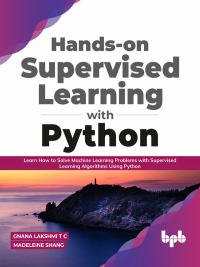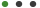# Hands-on Supervised Learning with Python

Formats - EPUB, PDF

Pages - 384

ISBN - 9789389328974

Tags - Best Seller#### Description

Hands-On ML problem solving and creating solutions using Python.

## Key Features

Introduction to Python Programming
Python for Machine Learning
Introduction to Machine Learning
Introduction to Predictive Modelling, Supervised and Unsupervised Algorithms
Linear Regression, Logistic Regression and Support Vector Machines

## Description

You will learn about the fundamentals of Machine Learning and Python programming post, which you will be introduced to predictive modelling and the different methodologies in predictive modelling. You will be introduced to Supervised Learning algorithms and Unsupervised Learning algorithms and the difference between them.
We will focus on learning supervised machine learning algorithms covering Linear Regression, Logistic Regression, Support Vector Machines, Decision Trees and Artificial Neural Networks. For each of these algorithms, you will work hands-on with open-source datasets and use python programming to program the machine learning algorithms. You will learn about cleaning the data and optimizing the features to get the best results out of your machine learning model. You will learn about the various parameters that determine the accuracy of your model and how you can tune your model based on the reflection of these parameters.

## What will you learn

Get a clear vision of what is Machine Learning and get familiar with the foundation principles of Machine learning.
Understand the Python language-specific libraries available for Machine learning and be able to work with those libraries.
Explore the different Supervised Learning based algorithms in Machine Learning and know how to implement them when a real-time use case is presented to you.
Have hands-on with Data Exploration, Data Cleaning, Data Preprocessing and Model implementation.
Get to know the basics of Deep Learning and some interesting algorithms in this space.
Choose the right model based on your problem statement and work with EDA techniques to get good accuracy on your model

## Who this book is for

This book is for anyone interested in understanding Machine Learning. Beginners, Machine Learning Engineers and Data Scientists who want to get familiar with Supervised Learning algorithms will find this book helpful.

1. Introduction to Python Programming
2. Python for Machine Learning
3. Introduction to Machine Learning
4. Supervised Learning and Unsupervised Learning
5. Linear Regression: A Hands-on guide
6. Logistic Regression – An Introduction
7. A sneak peek into the working of Support Vector machines(SVM)
8. Decision Trees
9. Random Forests
10. Time Series models in Machine Learning
11. Introduction to Neural Networks
12. Recurrent Neural Networks
13. Convolutional Neural Networks
14. Performance Metrics
15. Introduction to Design Thinking
16. Design Thinking Case Study

No Datials Available